SPSS TUTORIALS BASICS ANOVA REGRESSION FACTOR CORRELATION

# SPSS – Delete Selection of Variables with Python

Multivariate analyses are often plagued by missing values. A common strategy is to first remove any variables and cases with high percentages of missingness. We then sometimes use pairwise deletion of missing values or impute them.
Now, for finding variables with many missing values, you could

• ensure you specify user missing values;
• run a simple DESCRIPTIVES table;
• inspect which variables have relatively low N;
• type out a DELETE VARIABLES command containing those variable names and run it.

Now, if you've a vast number of variables or you run these steps routinely on different files, you might want to try and automate them. This lesson shows how to do so on many-missings.sav, part of which is shown below.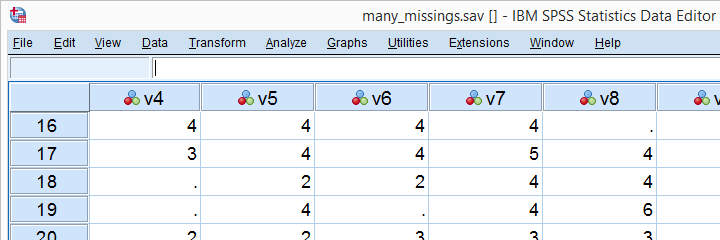## Inspect Case Count

A very simple way to find the number of cases in our data -ignoring any WEIGHT, FILTER or SPLIT FILE setting- is moving to the last case by pressing ctrl and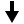and inspecting its case number.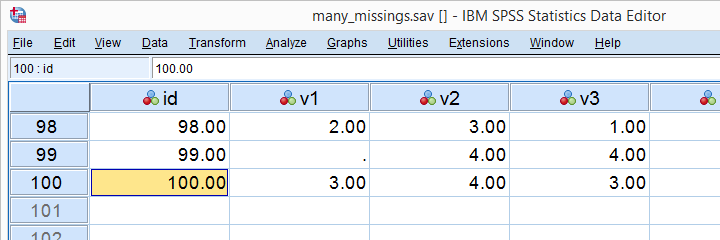Our data contain 100 cases. By the way, an alternative way to obtain this number is to run SHOW N.

## Run Basic Descriptives Table

For the data at hand, user missing values have already been set. We'll now follow part of our non-Python approach and run a basic descriptives table with DESCRIPTIVES v1 TO v20. Since we've 100 cases, (100 - N) is the number of missing values in each variable. We'll keep a screenshot of this table in Irfanview or some other program.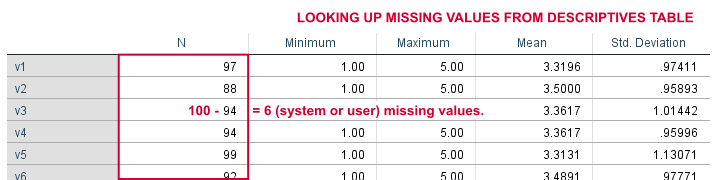## Look Up All Values in Single Variable

We'll first simply look up all values in our first variable, v1, with the syntax below.

*Look up all data values in variable.

begin program python3.
import spssdata
with spssdata.Spssdata("v1") as allData: # contains all values for v1
for case in allData: # for the 100 cases in our data
print(case)
end program.

## Result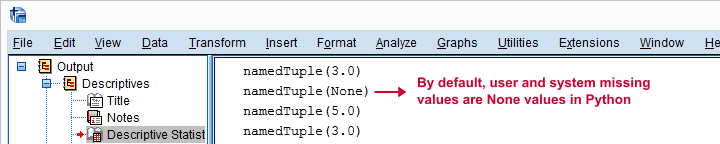First off, Python returns each case as a namedTuple because it may hold several values. In this case it doesn't because we specified just one variable. We can extract the first -and only- value from a tuple with `case` as we'll do in a minute.
Second, both system and user missing values result in None by default. We can thus obtain the number of missing values for a variable by counting the number of None values it holds. We'll do just that for v2 in the next syntax example.

## Count None Values in Single Variable

*Count (system and user) missing values in variable.

begin program python3.
import spssdata
with spssdata.Spssdata("v2") as allData:
misCnt = 0 # missing values is 0 at first
for case in allData:
if case is None: # system or user missing value
misCnt += 1 # add 1 to missing values
print(misCnt)
end program.

This returns 12. Our DESCRIPTIVES table confirms that v2 has (100 - 12 = ) 88 valid values.

## Expand Range of Variables

You probably don't always want to loop over all variables but -rather- a range of adjacent variables specified with TO. In this case we'll have Python expand `v1 TO v20` with the syntax below. This returns a Python list object of variable names that we'll loop over in a minute.

*Expand range of variables.

begin program python3.
import spssaux
sDict = spssaux.VariableDict(caseless = True) # allow wrong casing for variable names
varList = sDict.expand("v1 to v20")
print(varList)
end program.

## Find Missing Values for Each Variable

After creating our list of target variables, we'll loop over it and count the None values in each. Like so, the syntax below basically combines the pieces of syntax we presented so far in this lesson. We'll first just print each variable name and its number of missing values. Again, we can verify the entire result with our DESCRIPTIVES table.

*Report number of missings in each variable.

begin program python3.
import spssaux,spssdata
sDict = spssaux.VariableDict(caseless = True)
varList = sDict.expand("v1 to v20")
for var in varList: # loop over v1 to v20
with spssdata.Spssdata(var) as allData:
misCnt = 0 # = count of missing values per variable
for case in allData:
if case is None: # = missing value
misCnt += 1 # add 1 to counter
print(var,misCnt) # verify with DESCRIPTIVES table
end program.

## Result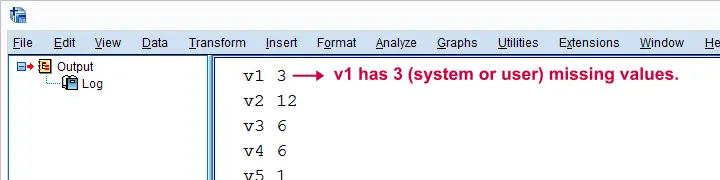## Create SPSS DELETE VARIABLES Syntax

We've chosen to delete all variables holding 15 or more missing values. We'll create a Python string called spssSyntax that initially just holds DELETE VARIABLES. We'll then concatenate each variable for which `misCnt >= 15` and a space to it. Last, we'll add a period to our SPSS command and inspect it.

*Create and inspect syntax.

begin program python3.
import spssaux,spssdata,spss
spssSyntax = "DELETE VARIABLES "
sDict = spssaux.VariableDict(caseless = True)
varList = sDict.expand("v1 to v20")
for var in varList:
with spssdata.Spssdata(var) as allData:
misCnt = 0
for case in allData:
if case == None:
misCnt += 1
if misCnt >= 15: # variable has at least 15 missing values
spssSyntax += var + ' ' # add variable name to DELETE VARIABLES
print(spssSyntax + ".") # add period to command
end program.

## Delete Variables

Since our DELETE VARIABLES command looks great, we're basically there; we'll comment-out the `print` statement and replace it with `spss.Submit` for having Python run our SPSS syntax. Since this requires the spss module, we need to add it to our `import` command (line 4, below).

*Create and run syntax.

begin program python3.
import spssaux,spssdata,spss
spssSyntax = "DELETE VARIABLES "
sDict = spssaux.VariableDict(caseless = True)
varList = sDict.expand("v1 to v20")
for var in varList:
with spssdata.Spssdata(var) as allData:
misCnt = 0
for case in allData:
if case is None:
misCnt += 1
if misCnt >= 15:
spssSyntax += var + ' '
#print spssSyntax + "."
spss.Submit(spssSyntax + ".")
end program.

## Final Notes

That's it. Note that we could modify this syntax to remove constants (variables for which each case has the same value) from our data too. Or even variables with low variance. The basic limitation here is that any WEIGHT, FILTER or SPLIT FILE will be ignored but this is rarely an issue.